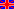http://www.wobsta.de/uni/tippetop/friction.shtml.en Tippe top: Necessity of friction »«Christian Friedl, André Wobst, 2005/05/25, 05:40 PMContentsModelFrictionElasticityMysteryExplanationPlotsAnimationInformations

# Necessity of frictionWe must consider the forces of friction, although they complicate our affair in important manner. This can be seen as follows:

During the motion the center of mass moves up. This implies an increasing of the potential energy. But in the same way we have a decreasing of the kinetic energy. At the beginning and the end of the simulation the symmetry axis is perpendicular to the underground. So we have a dimination of the vertical oriented angular momentum. This is only possible if there is an vertical torque.

The vector of the gravitational force already shows downside, so it gives no contribution to a vertical torque (note the 'right-hand-rule' to determine the cross-product). The only force which is able to produce the required torque is the friction, so we have to take the friction force into account.

to the next section: Elasticity

 Homepage  |  Theoretical physics I  |  Physics department  |  Augsburg University